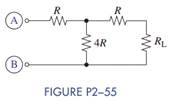Chat Now

# Select the value of R in Figure P2−55 so that R AB = R L .

### Select the value of R in Figure P2−55 so that R AB = R L .

Select the value of R in Figure P2−55 so that RAB = RL.manish jayant 28-Jul-2020Get solution